13.1 Sequences and their notations  (Page 7/15)

 Page 7 / 15

$\left(\frac{12}{6}\right)!$

$\frac{12!}{6!}$

$665,280$

$\frac{100!}{99!}$

For the following exercises, write the first four terms of the sequence.

${a}_{n}=\frac{n!}{{n}^{\text{2}}}$

First four terms: $1,\frac{1}{2},\frac{2}{3},\frac{3}{2}$

${a}_{n}=\frac{3\cdot n!}{4\cdot n!}$

${a}_{n}=\frac{n!}{{n}^{2}-n-1}$

First four terms: $-1,2,\frac{6}{5},\frac{24}{11}$

${a}_{n}=\frac{100\cdot n}{n\left(n-1\right)!}$

Graphical

For the following exercises, graph the first five terms of the indicated sequence

${a}_{n}=\frac{{\left(-1\right)}^{n}}{n}+n$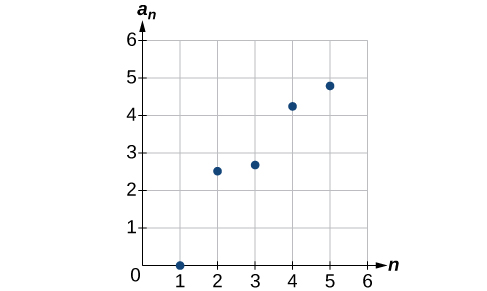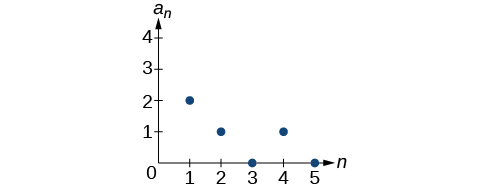${a}_{n}=\frac{\left(n+1\right)!}{\left(n-1\right)!}$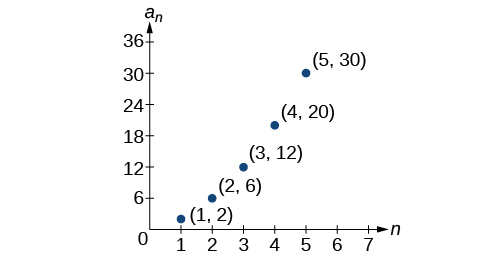For the following exercises, write an explicit formula for the sequence using the first five points shown on the graph.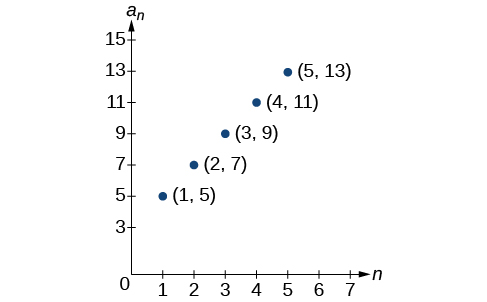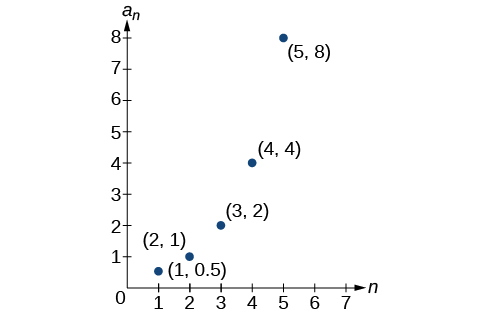${a}_{n}={2}^{n-2}$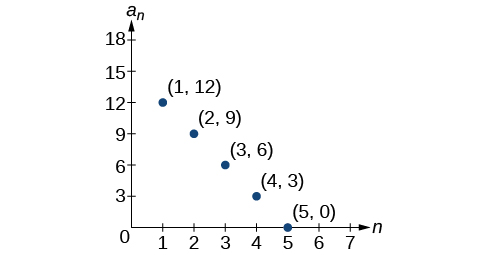For the following exercises, write a recursive formula for the sequence using the first five points shown on the graph.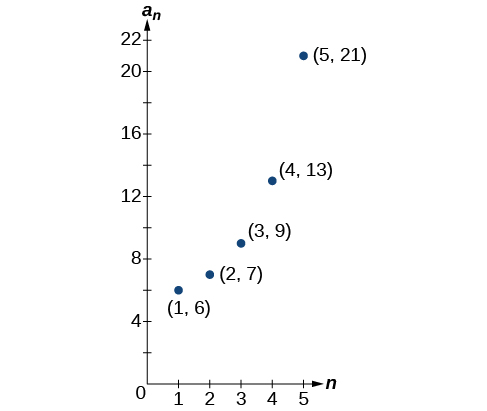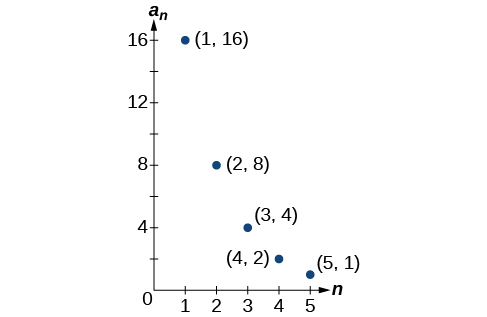Technology

Follow these steps to evaluate a sequence defined recursively using a graphing calculator:

• On the home screen, key in the value for the initial term $\text{\hspace{0.17em}}{a}_{1}\text{\hspace{0.17em}}$ and press [ENTER] .
• Enter the recursive formula by keying in all numerical values given in the formula, along with the key strokes [2ND] ANS for the previous term $\text{\hspace{0.17em}}{a}_{n-1}.\text{\hspace{0.17em}}$ Press [ENTER] .
• Continue pressing [ENTER] to calculate the values for each successive term.

For the following exercises, use the steps above to find the indicated term or terms for the sequence.

Find the first five terms of the sequence Use the> Frac feature to give fractional results.

First five terms: $\frac{29}{37},\frac{152}{111},\frac{716}{333},\frac{3188}{999},\frac{13724}{2997}$

Find the 15 th term of the sequence

Find the first five terms of the sequence

First five terms: $2,3,5,17,65537$

Find the first ten terms of the sequence

Find the tenth term of the sequence

${a}_{10}=7,257,600$

Follow these steps to evaluate a finite sequence defined by an explicit formula. Using a TI-84, do the following.

• In the home screen, press [2ND] LIST .
• Scroll over to OPS and choose “seq(” from the dropdown list. Press [ENTER] .
• In the line headed “Expr:” type in the explicit formula, using the $\text{\hspace{0.17em}}\left[\text{X,T},\theta ,n\right]\text{\hspace{0.17em}}$ button for $\text{\hspace{0.17em}}n$
• In the line headed “Variable:” type in the variable used on the previous step.
• In the line headed “start:” key in the value of $\text{\hspace{0.17em}}n\text{\hspace{0.17em}}$ that begins the sequence.
• In the line headed “end:” key in the value of $\text{\hspace{0.17em}}n\text{\hspace{0.17em}}$ that ends the sequence.
• Press [ENTER] 3 times to return to the home screen. You will see the sequence syntax on the screen. Press [ENTER] to see the list of terms for the finite sequence defined. Use the right arrow key to scroll through the list of terms.

Using a TI-83, do the following.

• In the home screen, press [2ND] LIST .
• Scroll over to OPS and choose “seq(” from the dropdown list. Press [ENTER] .
• Enter the items in the order “Expr” , “Variable” , “start” , “end” separated by commas. See the instructions above for the description of each item.
• Press [ENTER] to see the list of terms for the finite sequence defined. Use the right arrow key to scroll through the list of terms.

For the following exercises, use the steps above to find the indicated terms for the sequence. Round to the nearest thousandth when necessary.

List the first five terms of the sequence ${a}_{n}=-\frac{28}{9}n+\frac{5}{3}.$

List the first six terms of the sequence

First six terms: $0.042,0.146,0.875,2.385,4.708$

List the first five terms of the sequence ${a}_{n}=\frac{15n\cdot {\left(-2\right)}^{n-1}}{47}$

List the first four terms of the sequence ${a}_{n}={5.7}^{n}+0.275\left(n-1\right)!$

First four terms: $5.975,32.765,185.743,1057.25,6023.521$

List the first six terms of the sequence ${a}_{n}=\frac{n!}{n}.$

Extensions

Consider the sequence defined by ${a}_{n}=-6-8n.$ Is ${a}_{n}=-421$ a term in the sequence? Verify the result.

If $\text{\hspace{0.17em}}{a}_{n}=-421\text{\hspace{0.17em}}$ is a term in the sequence, then solving the equation $-421=-6-8n$ for $n$ will yield a non-negative integer. However, if $\text{\hspace{0.17em}}-421=-6-8n,\text{\hspace{0.17em}}$ then $n=51.875$ so ${a}_{n}=-421$ is not a term in the sequence.

What term in the sequence ${a}_{n}=\frac{{n}^{2}+4n+4}{2\left(n+2\right)}$ has the value $41?$ Verify the result.

Find a recursive formula for the sequence ( Hint : find a pattern for $\text{\hspace{0.17em}}{a}_{n}\text{\hspace{0.17em}}$ based on the first two terms.)

${a}_{1}=1,{a}_{2}=0,{a}_{n}={a}_{n-1}-{a}_{n-2}$

Calculate the first eight terms of the sequences ${a}_{n}=\frac{\left(n+2\right)!}{\left(n-1\right)!}$ and ${b}_{n}={n}^{3}+3{n}^{2}+2n,$ and then make a conjecture about the relationship between these two sequences.

Prove the conjecture made in the preceding exercise.

$\frac{\left(n+2\right)!}{\left(n-1\right)!}=\frac{\left(n+2\right)·\left(n+1\right)·\left(n\right)·\left(n-1\right)·...·3·2·1}{\left(n-1\right)·...·3·2·1}=n\left(n+1\right)\left(n+2\right)={n}^{3}+3{n}^{2}+2n$

A laser rangefinder is locked on a comet approaching Earth. The distance g(x), in kilometers, of the comet after x days, for x in the interval 0 to 30 days, is given by g(x)=250,000csc(π30x). Graph g(x) on the interval [0, 35]. Evaluate g(5)  and interpret the information. What is the minimum distance between the comet and Earth? When does this occur? To which constant in the equation does this correspond? Find and discuss the meaning of any vertical asymptotes.
The sequence is {1,-1,1-1.....} has
how can we solve this problem
Sin(A+B) = sinBcosA+cosBsinA
Prove it
Eseka
Eseka
hi
Joel
June needs 45 gallons of punch. 2 different coolers. Bigger cooler is 5 times as large as smaller cooler. How many gallons in each cooler?
7.5 and 37.5
Nando
find the sum of 28th term of the AP 3+10+17+---------
I think you should say "28 terms" instead of "28th term"
Vedant
the 28th term is 175
Nando
192
Kenneth
if sequence sn is a such that sn>0 for all n and lim sn=0than prove that lim (s1 s2............ sn) ke hole power n =n
write down the polynomial function with root 1/3,2,-3 with solution
if A and B are subspaces of V prove that (A+B)/B=A/(A-B)
write down the value of each of the following in surd form a)cos(-65°) b)sin(-180°)c)tan(225°)d)tan(135°)
Prove that (sinA/1-cosA - 1-cosA/sinA) (cosA/1-sinA - 1-sinA/cosA) = 4
what is the answer to dividing negative index
In a triangle ABC prove that. (b+c)cosA+(c+a)cosB+(a+b)cisC=a+b+c.
give me the waec 2019 questionsByByBy Nick Swain